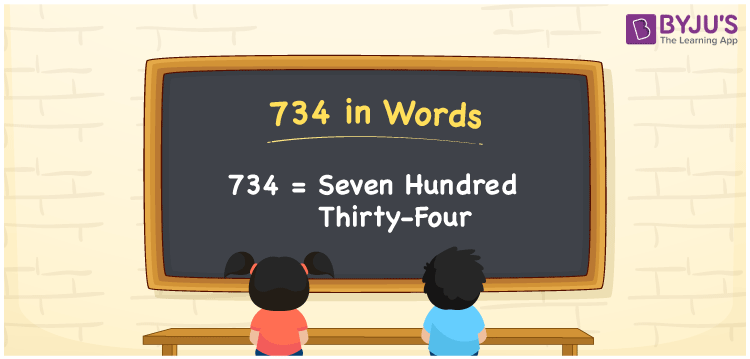# 734 in Words

734 in words is “Seven hundred thirty-four”. The number 734 has three digits and its place value helps to write the number name. The number 734 appears between 733 and 735. To convert the number 734 into words, we use the place value system. Now, let us have a look at the process of writing the number 734 in words in this article.

 734 in Words: Seven Hundred Thirty-four. Seven Hundred Thirty-four in Numerical Form: 734.

## 734 in English Words## How to Write 734 in Words?

The place value table for the natural number 734 is given below:

 Hundreds Tens Ones 7 3 4

The expanded form of 734 is as follows:

= 7 × Hundred + 3 × Ten + 4 × One

= 7 × 100 + 3 × 10 + 4 × 1

= 700 + 30 + 4

= 734

= seven hundred thirty-four

Hence, 734 in words is seven hundred thirty-four.

734 in words – Seven hundred thirty-four

Is 734 an odd number? – No

Is 734 an even number? – Yes

Is 734 a perfect square number? – No

Is 734 a perfect cube number? – No

Is 734 a prime number? – No

Is 734 a composite number? – Yes

## Frequently Asked Questions on 734 in Words

Q1

### Write 734 in words.

734 in words is seven hundred thirty-four.

Q2

### Simplify 700 + 34, and express it in words.

Simplifying 700 + 34, we get 734. Hence, 734 in words is seven hundred thirty-four.

Q3

### Is 734 a composite number?

Yes, 734 is a composite number.